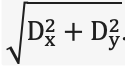Spitzer Documentation & Tools
IRAC Instrument Handbook

### C.3.8   Pixel Phase

As in 4.6, we define pixel phase as the offset between the centroid of a stellar image and the center of the pixel in which that centroid lies. For example, an object whose centroid has pixel coordinates 128.23, 127.85 has a pixel phase of Dx,Dy = (–0.27, 0.35). The pixel phase effect is here characterized in terms of the radial pixel phase p =. To shift a 5 × 5 oversampled  PRF to a given pixel phase we have adopted the following technique:

i) Magnify the PRFs by a large factor, e.g. 20, using linear interpolation (so the resultant PRF sampling is x100).

ii) Re-center the PRF by shifting it to its centroid. (Note that the estimate of the centroid of a source is itself a function of the method used to determine the centroid, so ideally you would use equivalent algorithms to find centroids in the (C)BCDs as you do to centroid the PRF. Note also that some of the IDL centroiding functions perform poorly with the very undersampled IRAC data at 3.6 and 4.5 µm).

ii) Shift the PRF array (in the example above a shift by 27, –35 resampled pixels would move the desired PRF to the center of the array).

iii) Extract the point source realization by sampling the PRF at intervals corresponding to native pixel increments (every 100 oversampled pixels in this example), making sure to pick up the center of the central pixel.

In IDL the commands would be:

i) Use rebin on the 1282, 5x oversampled PRF to produce the magnified PRF:
magPRF = rebin(PRF,2560,2560).

ii) Re-center the PRF. We find that calculating the first moments is usually a robust way to find the centroids. Set xmin, xmax, and ymin and ymax to approximately the same values in native pixels as you use to estimate the centroids in your data:

xx=float(lindgen(2560,2560)mod2560)
yy=float(lindgen(2560,2560)/2560)

xcen=total(xx[xmin:xmax,ymin:ymax]*magPRF[xmin:xmax,ymin:ymax])/total(magPRF[xmin:xmax,ymin:ymax])

ycen=total(yy[xmin:xmax,ymin:ymax]*magPRF[xmin:xmax,ymin:ymax])/total(magPRF[xmin:xmax,ymin:ymax])

xcensh=nx/2-round(xcen)
ycensh=ny/2-round(ycen)
cenPRF = shift(magPRF,xcensh,ycensh)

ii) Shift the re-centered PRF to the center of the central PRF pixel and trim to an integer multiple of the 100x oversampling factor such that the central pixel (1280,1280) is moved to (1200,1200), the center of the trimmed array:

shiftedPRF=shift(cenPRF,27,-35)
phasedPRF=fltarr(2500,2500)
trimmedPRF=shiftedPRF[80:2559,80:2559]
phasedPRF[0:2479,0:2479]=trimmedPRF

iii) Sample the trimmed PRF to produce the point source realization:

PSR = rebin(trimmedPRF,25,25,/SAMPLE)

The center of the zero phase PSR in this example should be IDL pixel (12,12).